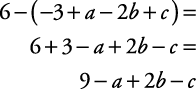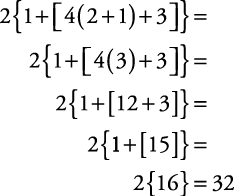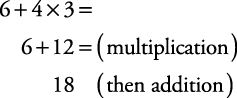## Grouping Symbols

There are basically three types of grouping symbols: parentheses, brackets, and braces.

#### Parentheses ( )

Parentheses are used to group numbers or variables. Everything inside parentheses must be done before any other operations.

##### Example 1

Simplify.

50(2 + 6) = 50(8) = 400

When a parenthesis is preceded by a minus sign, to remove the parentheses, change the sign of each term within the parentheses.

##### Example 2

Simplify.#### Brackets [ ] and braces { }

Brackets and braces also are used to group numbers or variables. Technically, they are used after parentheses. Parentheses are to be used first, then brackets, and then braces: {[( )]}. Sometimes, instead of brackets or braces, you will see the use of larger parentheses.

((3 + 4) · 5) + 2

A number using all three grouping symbols would look like this:

2{1 + [4(2 + 1) + 3]}

##### Example 3

Simplify 2{1 + [4(2 + 1) + 3]}. Notice that you work from the inside out.#### Order of operations

If multiplication, division, powers, addition, parentheses, and so forth are all contained in one problem, the order of operations is as follows:

1. Parentheses

3. Multiplication or division (in the order it occurs from left to right)

4. Addition or subtraction (in the order it occurs from left to right)

Many students find the made up word PEMDAS helpful as a memory tool. The “P” reminds you that “parentheses” are done first; the “E” reminds you that “exponents” are done next; the “MD” reminds you to “multiply or divide” in the order it occurs from left to right; and the “AS” reminds you to “add or subtract” in the order it occurs from left to right.

Also, some students remember the order using the following phrase:

 Please Excuse My Dear Aunt Sally Parantheses Exponents Multiply or Divide Add or Subtract
##### Example 4

Simplify the following problems.

1.2.